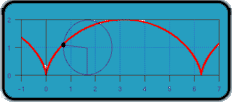All about flooble | fun stuff | Get a free chatterbox | Free JavaScript | Avatarsperplexus dot infoThe wheels on the bus go.. (Posted on 2003-06-23)If you drew a dot on the edge of a wheel and traced the path of the dot as the wheel rolled one complete revolution along a line, then the path formed would be called a cycloid (shown below), combining both forward and circular motion.If a wheel of radius 1 traces out such a path, what is the length of the path formed by one complete revolution?

 See The Solution Submitted by DJ Rating: 4.3571 (14 votes)Comments: ( Back to comment list | You must be logged in to post comments.)half way there| Comment 7 of 9 |Taking an approach similar to friedlinguini, I considered the motion to be a combination of movement around a circle clockwise starting from the bottom and translation in the x direction. So in the y direction there is no contribution from the horizontal translation.
call the angle t (as in theta)
dy= sin(t) dt
dx= (1-cos(t)) dt
the arc length S is found by integrating ds
ds^2=(dx^2+dy^2)=sin^2 + 1 + cos^2 -2cos=2(1-cos(t))
so S=the integral from 0 to 2π of √[2(1-cos(t))] dt

which is where I got stuck
but friedlinguini is correct
the arclength is 8
the horizontal distance travelled is 2π
 Posted by Larry on 2004-02-21 14:26:04Please log in:

 Search: Search body:
Forums (2)